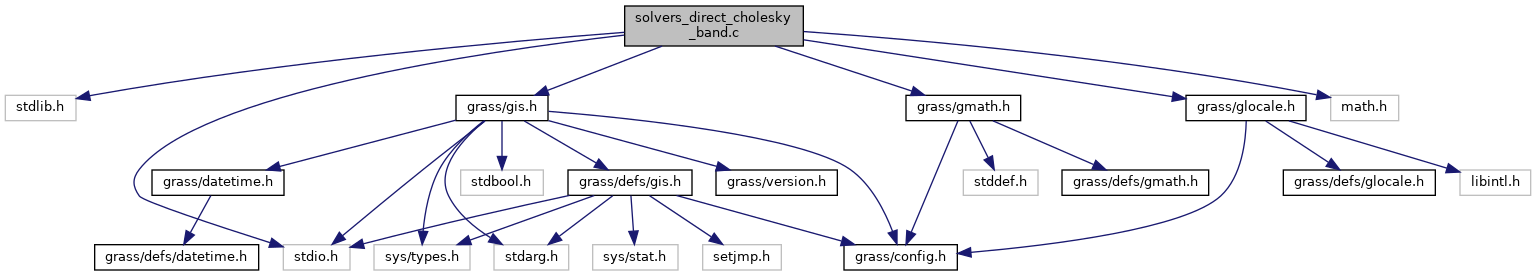GRASS GIS 7 Programmer's Manual  7.9.dev(2021)-e5379bbd7
solvers_direct_cholesky_band.c File Reference
`#include <stdlib.h>`
`#include <stdio.h>`
`#include <math.h>`
`#include <grass/gis.h>`
`#include <grass/gmath.h>`
`#include <grass/glocale.h>`
Include dependency graph for solvers_direct_cholesky_band.c:Go to the source code of this file.

## Functions

void G_math_cholesky_sband_decomposition (double **A, double **T, int rows, int bandwidth)
Cholesky decomposition of a symmetric band matrix. More...

void G_math_solver_cholesky_sband (double **A, double *x, double *b, int rows, int bandwidth)
Cholesky symmetric band matrix solver for linear equation systems of type Ax = b. More...

void G_math_cholesky_sband_substitution (double **T, double *x, double *b, int rows, int bandwidth)
Forward and backward substitution of a lower tringular symmetric band matrix of A from system Ax = b. More...

void G_math_cholesky_sband_invert (double **A, double *invAdiag, int rows, int bandwidth)

void G_math_solver_cholesky_sband_invert (double **A, double *x, double *b, double *invAdiag, int rows, int bandwidth)

## ◆ G_math_cholesky_sband_decomposition()

 void G_math_cholesky_sband_decomposition ( double ** A, double ** T, int rows, int bandwidth )

Cholesky decomposition of a symmetric band matrix.

Parameters
 A (double**) the input symmetric band matrix T (double**) the resulting lower tringular symmetric band matrix rows (int) number of rows bandwidth (int) the bandwidth of the symmetric band matrix

Definition at line 18 of file solvers_direct_cholesky_band.c.

## ◆ G_math_cholesky_sband_invert()

 void G_math_cholesky_sband_invert ( double ** A, double * invAdiag, int rows, int bandwidth )

Definition at line 121 of file solvers_direct_cholesky_band.c.

## ◆ G_math_cholesky_sband_substitution()

 void G_math_cholesky_sband_substitution ( double ** T, double * x, double * b, int rows, int bandwidth )

Forward and backward substitution of a lower tringular symmetric band matrix of A from system Ax = b.

Parameters
 T (double**) the lower triangle symmetric band matrix x (double*) the resulting vector b (double*) the right hand side of Ax = b rows (int) number of rows bandwidth (int) the bandwidth of the symmetric band matrix

Definition at line 87 of file solvers_direct_cholesky_band.c.

## ◆ G_math_solver_cholesky_sband()

 void G_math_solver_cholesky_sband ( double ** A, double * x, double * b, int rows, int bandwidth )

Cholesky symmetric band matrix solver for linear equation systems of type Ax = b.

Parameters
 A (double**) the input symmetric band matrix x (double*) the resulting vector, result is written in here b (double*) the right hand side of Ax = b rows (int) number of rows bandwidth (int) the bandwidth of the symmetric band matrix

Definition at line 61 of file solvers_direct_cholesky_band.c.

## ◆ G_math_solver_cholesky_sband_invert()

 void G_math_solver_cholesky_sband_invert ( double ** A, double * x, double * b, double * invAdiag, int rows, int bandwidth )

Definition at line 165 of file solvers_direct_cholesky_band.c.